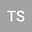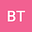Dynamic transitions and bifurcations of 1D reaction-diffusion equations: The Self-adjoint case
••Burhan Tiryakioglu
Marmara University
Author ProfileAbstract

This paper deals with the classification of transition phenomena in the most basic dissipative system possible, namely the 1D reaction diffusion equation. The emphasis is on the relation between the linear and nonlinear terms and the effect of the boundaries which influence the first transitions. We consider the cases where the linear part is self-adjoint with 2nd order and 4th order derivatives which is the case which most often arises in applications. We assume that the nonlinear term depends on the function and its first derivative which is basically the semilinear case for the second order reaction-diffusion system. As for the boundary conditions, we consider the typical Dirichlet, Neumann and periodic boundary settings. In all the cases, the equations admit a trivial steady state which loses stability at a critical parameter. We aim to classify all possible transitions and bifurcations that take place. Our analysis shows that these systems display all three types of transitions: continuous, jump and mixed and display transcritical, supercritical bifurcations with bifurcated states such as finite equilibria, circle of equilibria, and slowly rotating limit cycle. Many applications found in the literature are basically corollaries of our main results. We apply our results to classify the first transitions of the Chaffee-Infante equation, the Fisher-KPP equation, the Kuramoto Sivashinsky equation and the Swift-Hohenberg equation.

Peer review status:ACCEPTED

11 Sep 2021Submitted to Mathematical Methods in the Applied Sciences
12 Sep 2021Submission Checks Completed
12 Sep 2021Assigned to Editor
05 Oct 2021Reviewer(s) Assigned
05 Oct 2021Review(s) Completed, Editorial Evaluation Pending
06 Oct 2021Editorial Decision: Revise Major